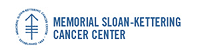## Memorial Sloan-Kettering Cancer Center, Dept. of Epidemiology & Biostatistics Working Paper Series

#### Abstract

The area under the curve (AUC) is the most common statistical approach to evaluate the discriminatory power of a set of factors in a binary regression model. A nested model framework is used to ascertain whether the AUC increases when new factors enter the model. Two statistical tests are proposed for the difference in the AUC parameters from these nested models. The asymptotic null distributions for the two test statistics are derived from the scenarios: (A) the difference in the AUC parameters is zero and the new factors are not associated with the binary outcome, (B) the difference in the AUC parameters is less than a strictly positive value. A confidence interval for the difference in AUC parameters is developed. Simulations are generated to determine the finite sample operating characteristics of the tests and a pancreatic cancer data example is used to illustrate this approach.

Biostatistics

COinS